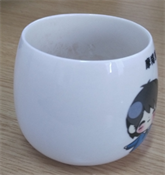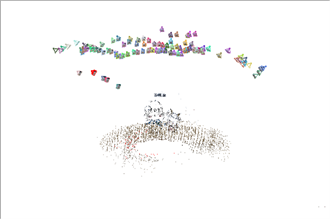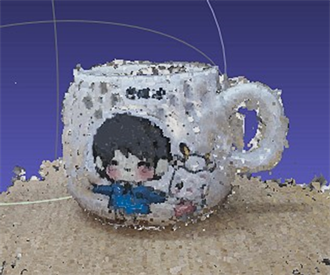﻿ 基于非量测相片的三维模型重建

# 基于非量测相片的三维模型重建Three-Dimensional Model Reconstruction Based on Non-Measured Photo

Abstract: With the development of science, the requirement of visual feeling is improved, an increasing number of three-dimensional image products enter life. Nevertheless, the three-dimensional model reconstruction is subject to many restrictions, and does not meet the public requirement. In recent years, three-dimensional reconstruction with non-measurement photos has become one of the popular ways of 3D model reconstruction. This paper mainly introduces the process of 3D model reconstruction, including the use of non-measurement photos for camera calibration, extraction and matching of feature points, line constraint and intrinsic matrix calculation, three-dimensional reconstruction, error analysis. Lastly, the paper briefly analyzes the prospect of this method.

1. 引言

2. 基于相片的三维几何信息计算方法

2.1. 相机标定

2.1.1. 坐标系转换

2.1.2. 内参提取

$Z\left[\begin{array}{c}x\\ y\\ 1\end{array}\right]=\left[\begin{array}{ccc}f& 0& {c}_{x}\\ 0& f& {c}_{y}\\ 0& 0& 1\end{array}\right]\left[\begin{array}{c}X\\ Y\\ Z\end{array}\right]$ (1)

2.1.3. 外参提取

$sx=K\left[\begin{array}{cc}R& T\end{array}\right]\left[\begin{array}{c}X\\ 1\end{array}\right]$ (2)

2.2. 特征点提取与匹配

2.3. 极线约束与本征矩阵

$0={{x}^{\prime }}_{2}E{{x}^{\prime }}_{1}$ (3)

$\begin{array}{l}{{x}^{\prime }}_{1}={K}^{-1}{x}_{1}\\ {{x}^{\prime }}_{2}={K}^{-1}{x}_{2}\\ E={\stackrel{⌢}{T}}_{2}{R}_{2}\end{array}$ (4)

3. 三维重建方法

3.1. 双目重建

$s{x}_{2}=K\left(RX+T\right)$ (5)

$\stackrel{⌢}{x}K\left[\begin{array}{cc}R& T\end{array}\right]\left[\begin{array}{c}X\\ 1\end{array}\right]=0$ (6)

3.2. 多目重建

OpenCV中拥有solvePnP和solvePnPRansac函数，只需知道空间中部分点的坐标和其对应点的像素坐标，就可以通过solvePnP计算获得相机的空间坐标。进行多目重建的过程原理是首先对最初的两张相片进行双目重建，获得了空间中的一些点，然后加入第三张相片，令此相片与第二张相片进行特征点的匹配，在形成的匹配点中包含相片二与相片一之间的部分匹配点，同时在第三张相片中这些点的像素坐标已知，即可通过solvePnP得到第三个相机的空间位置，得到相机三到相机一的变换矩阵。

4. 实验与分析

4.1. 数据准备

4.2. 三维重建结果Figure 1. Flow chart of multi-purpose reconstructionFigure 2. Two-dimensional non-measuring photoFigure 3. Sparse reconstructionFigure 4. 3D reconstruction of the image

5. 结束语

 李秀全, 陈竹安, 张立亭. 非量测相机影像三维模型构建及精度检验[J]. 测绘科学, 2016(6): 144-147.

 郑顺义, 张祖勋, 翟瑞芳. 基于非量测相机的复杂物体三维重建[J]. 武汉大学学报(信息科学版), 2008(5): 446- 449.

 李天子, 郭辉. 非量测数码相机的影像纠正[J]. 测绘通报, 2006(10): 59-61.

 郑逢杰, 余涛, 袁国体, 等. 相机几何标定方法综述[J]. 太原科技, 2010(2): 72-73.

 时愈, 罗枭, 邓哲煜, 等. 基于多观测点图像SURF特征配准及去模糊的三维重建[J]. 光学与光电技术, 2016(1): 28-32.

 Lepetit, V., Moreno-Noguer, F. and Fua, P. (2009) EPnP: An Accurate O(n) Solution to the PnP Problem. International Journal of Computer Vision, 81, 155-166.

 季顺平, 史云. 车载全景相机的影像匹配和光束法平差[J]. 测绘学报, 2013, 42(1): 94-100.

 张正鹏, 江万寿, 张靖. 光流特征聚类的车载全景序列影像匹配方法[J]. 测绘学报, 2014, 43(12): 1266-1273.

 徐芳, 梅文胜, 张利. 线阵全景相机检校模型研究[J]. 武汉大学学报(信息科学版), 2014, 39(4):417-421.

 张雯, 程亮, 张群, 等. 三维模型几何质量评价方法[J]. 测绘通报, 2014(7): 44-47.

Top